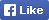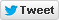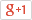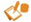Computer/Electronics Questions and Answers
C, C++, VC++, COM/DCOM, DLL and moreLoginSign upHome Electronics Computer Questions Member's Area News Site Map About

Call by value: In call by value method, the called function creates a new set of variables and copies the values of arguments into them.
Example: Program showing Call by value method

```void swap(int x, int y)
{
int temp;
temp = x;
x = y;
y = temp;
printf("Swapped values are a = %d and b = %d", x, y);
}

void main()
{
int a = 7, b = 4;
swap(a, b);
printf("Original values are a = %d and b = %d", a, b);
printf("The values after swap are a = %d and b = %d", a, b);
}

Output:
Original Values are a = 7 and b = 4
Swapped values are a = 4 and b = 7

```
The values after swap are a = 7 and b = 4
This happens because when function swap() is invoked, the values of a and b gets copied on to x and y. The function actually swaps x and y while the original variables a and b remains intact.

Call by reference: In call by reference method, instead of passing a value to the function being called a reference/pointer to the original variable is passed.
Example: Program showing Call by reference method

```void swap(int *x, int *y)
{
int temp;
temp = *x;
*x = *y;
*y = temp;
printf("Swapped values are a = %d and b = %d", *x, *y);
}
void main()
{
int a = 7, b = 4;
swap(&a, &b);
printf("Original values are a = %d and b = %d",a,b);
printf("The values after swap are a = %d and b = %d",a,b);
}

Output:
Original Values are a = 7 and b = 4
Swapped values are a = 4 and b = 7
```
The values after swap are a = 4 and b = 7
This happens because when function swap() is invoked, it creates a reference for the first incoming integer a in x and the second incoming integer b in y.Discussion forum

Help us to improve this topic. Your feedback is essential to us. Please suggest your remarks, ratings and corrections regarding the above section. Please fill the form and submit. If your suggestion contains graphics/formatted text/word document, please attach file(s).

 Name: * Email: * Text Answer: * Attach Doc:

Note: Only members are allowed, * fields are mandatory.